# G.1   Introduction

G.1.1   This annex addresses the general question of obtaining from the estimate y of the measurand Y, and from the combined standard uncertainty uc(y) of that estimate, an expanded uncertainty Up = kpuc(y) that defines an interval y − Up ≤ Y ≤ y + Up that has a high, specified coverage probability or level of confidence p. It thus deals with the issue of determining the coverage factor kp that produces an interval about the measurement result y that may be expected to encompass a large, specified fraction p of the distribution of values that could reasonably be attributed to the measurand Y (see Clause 6).

G.1.2   In most practical measurement situations, the calculation of intervals having specified levels of confidence — indeed, the estimation of most individual uncertainty components in such situations — is at best only approximate. Even the experimental standard deviation of the mean of as many as 30 repeated observations of a quantity described by a normal distribution has itself an uncertainty of about 13 percent (see Table E.1 in Annex E).

In most cases, it does not make sense to try to distinguish between, for example, an interval having a level of confidence of 95 percent (one chance in 20 that the value of the measurand Y lies outside the interval) and either a 94 percent or 96 percent interval (1 chance in 17 and 25, respectively). Obtaining justifiable intervals with levels of confidence of 99 percent (1 chance in 100) and higher is especially difficult, even if it is assumed that no systematic effects have been overlooked, because so little information is generally available about the most extreme portions or “tails” of the probability distributions of the input quantities.

G.1.3   To obtain the value of the coverage factor kp that produces an interval corresponding to a specified level of confidence p requires detailed knowledge of the probability distribution characterized by the measurement result and its combined standard uncertainty. For example, for a quantity z described by a normal distribution with expectation μz and standard deviation σ, the value of kp that produces an interval μz ± kpσ that encompasses the fraction p of the distribution, and thus has a coverage probability or level of confidence p, can be readily calculated. Some examples are given in Table G.1.

Table G.1 — Value of the coverage factor kp that produces an interval having level of confidence p assuming a normal distribution
Level of confidence p Coverage factor kp
(percent)
68,27 1
90 1,645
95 1,960
95,45 2
99 2,576
99,73 3

NOTE   By contrast, if z is described by a rectangular probability distribution with expectation μz and standard deviation σ = a3‾‾, where a is the half‑width of the distribution, the level of confidence p is 57,74 percent for kp = 1; 95 percent for kp = 1,65; 99 percent for kp = 1,71; and 100 percent for kp ≥ 3‾‾ ≈ 1,73; the rectangular distribution is “narrower” than the normal distribution in the sense that it is of finite extent and has no “tails”.

G.1.4   If the probability distributions of the input quantities X1X2, ..., XN upon which the measurand Y depends are known [their expectations, variances, and higher moments (see C.2.13 and C.2.22) if the distributions are not normal distributions], and if Y is a linear function of the input quantities, Y = c1X1 + c2X2 + ... + cNXN, then the probability distribution of Y may be obtained by convolving the individual probability distributions . Values of kp that produce intervals corresponding to specified levels of confidence p may then be calculated from the resulting convolved distribution.

G.1.5   If the functional relationship between Y and its input quantities is nonlinear and a first‑order Taylor series expansion of the relationship is not an acceptable approximation (see 5.1.2 and 5.1.5), then the probability distribution of Y cannot be obtained by convolving the distributions of the input quantities. In such cases, other analytical or numerical methods are required.

G.1.6   In practice, because the parameters characterizing the probability distributions of input quantities are usually estimates, because it is unrealistic to expect that the level of confidence to be associated with a given interval can be known with a great deal of exactness, and because of the complexity of convolving probability distributions, such convolutions are rarely, if ever, implemented when intervals having specified levels of confidence need to be calculated. Instead, approximations are used that take advantage of the Central Limit Theorem.

# G.2   Central Limit Theorem

G.2.1   If Y = c1X1 + c2X2 + ... + cNXN = ∑Ni = 1ciXi and all the Xi are characterized by normal distributions, then the resulting convolved distribution of Y will also be normal. However, even if the distributions of the Xi are not normal, the distribution of Y may often be approximated by a normal distribution because of the Central Limit Theorem. This theorem states that the distribution of Y will be approximately normal with expectation E(Y) = ∑Ni = 1ciE(Xi) and variance σ2(Y ) = ∑Ni = 1c2iσ2(Xi), where E(Xi) is the expectation of Xi and σ2(Xi) is the variance of Xi, if the Xi are independent and σ2(Y ) is much larger than any single component c2iσ2(Xi) from a non‑normally distributed Xi.

G.2.2   The Central Limit Theorem is significant because it shows the very important role played by the variances of the probability distributions of the input quantities, compared with that played by the higher moments of the distributions, in determining the form of the resulting convolved distribution of Y. Further, it implies that the convolved distribution converges towards the normal distribution as the number of input quantities contributing to σ2(Y) increases; that the convergence will be more rapid the closer the values of c2iσ2(Xi) are to each other (equivalent in practice to each input estimate xi contributing a comparable uncertainty to the uncertainty of the estimate y of the measurand Y); and that the closer the distributions of the Xi are to being normal, the fewer Xi are required to yield a normal distribution for Y.

EXAMPLE   The rectangular distribution (see 4.3.7 and 4.4.5) is an extreme example of a non‑normal distribution, but the convolution of even as few as three such distributions of equal width is approximately normal. If the half‑width of each of the three rectangular distributions is a so that the variance of each is a23, the variance of the convolved distribution is σ2 = a2. The 95 percent and 99 percent intervals of the convolved distribution are defined by 1,937 σ and 2,379 σ, respectively, while the corresponding intervals for a normal distribution with the same standard deviation σ are defined by 1,960 σ and 2,576 σ (see Table G.1) .

NOTE 1   For every interval with a level of confidence p greater than about 91,7 percent, the value of kp for a normal distribution is larger than the corresponding value for the distribution resulting from the convolution of any number and size of rectangular distributions.

NOTE 2   It follows from the Central Limit Theorem that the probability distribution of the arithmetic mean q‾‾ of n observations qk of a random variable q with expectation μq and finite standard deviation σ approaches a normal distribution with mean μq and standard deviation σn‾‾ as n → ∞, whatever may be the probability distribution of q.

G.2.3   A practical consequence of the Central Limit Theorem is that when it can be established that its requirements are approximately met, in particular, if the combined standard uncertainty uc(y) is not dominated by a standard uncertainty component obtained from a Type A evaluation based on just a few observations, or by a standard uncertainty component obtained from a Type B evaluation based on an assumed rectangular distribution, a reasonable first approximation to calculating an expanded uncertainty Up = kpuc(y) that provides an interval with level of confidence p is to use for kp a value from the normal distribution. The values most commonly used for this purpose are given in Table G.1.

# G.3   The t‑distribution and degrees of freedom

G.3.1   To obtain a better approximation than simply using a value of kp from the normal distribution as in G.2.3, it must be recognized that the calculation of an interval having a specified level of confidence requires, not the distribution of the variable [Y − E(Y )]σ(Y ), but the distribution of the variable (y − Y )uc(y). This is because in practice, all that is usually available are y the estimate of Y as obtained from y = ∑Ni = 1cixi, where xi is the estimate of Xi; and the combined variance associated with y, u2c(y), evaluated from u2c(y) = ∑Ni = 1c2iu2(xi), where u(xi) is the standard uncertainty (estimated standard deviation) of the estimate xi.

NOTE   Strictly speaking, in the expression (y − Y )uc(y), Y should read E(Y ). For simplicity, such a distinction has only been made in a few places in this Guide. In general, the same symbol has been used for the physical quantity, the random variable that represents that quantity, and the expectation of that variable (see 4.1.1, notes).

G.3.2   If z is a normally distributed random variable with expectation μz and standard deviation σ, and z‾‾ is the arithmetic mean of n independent observations zk of z with s(z‾‾) the experimental standard deviation of z‾‾ [see Equations (3) and (5) in 4.2], then the distribution of the variable t = (z‾‾ − μz)s(z‾‾) is the t‑distribution or Student's distribution (C.3.8) with v = n − 1 degrees of freedom.

Consequently, if the measurand Y is simply a single normally distributed quantity X, Y = X; and if X is estimated by the arithmetic mean X‾‾‾ of n independent repeated observations Xk of X, with experimental standard deviation of the mean s(X‾‾‾), then the best estimate of Y is y = X‾‾‾ and the experimental standard deviation of that estimate is uc(y) = s(X‾‾‾). Then t = (z‾‾ − μz)s(z‾‾) = (X‾‾‾ − X)s(X‾‾‾) = (y − Y )uc(y) is distributed according to the t‑distribution with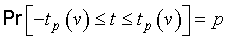(G.1a)
or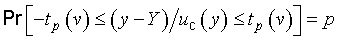(G.1b)
which can be rewritten as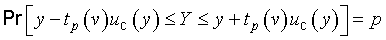(G.1c)

In these expressions, Pr[  ] means “probability of” and the t‑factor tp(v) is the value of t for a given value of the parameter v — the degrees of freedom (see G.3.3) — such that the fraction p of the t distribution is encompassed by the interval tp(v) to +tp(v). Thus the expanded uncertainty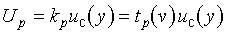(G.1d)
defines an interval y − Up to y + Up, conveniently written as Y = y ± Up, that may be expected to encompass a fraction p of the distribution of values that could reasonably be attributed to Y, and p is the coverage probability or level of confidence of the interval.

G.3.3   The degrees of freedom v is equal to n − 1 for a single quantity estimated by the arithmetic mean of n independent observations, as in G.3.2. If n independent observations are used to determine both the slope and intercept of a straight line by the method of least squares, the degrees of freedom of their respective standard uncertainties is v = n − 2. For a least-squares fit of m parameters to n data points, the degrees of freedom of the standard uncertainty of each parameter is v = n − m. (See Reference  for a further discussion of degrees of freedom.)

G.3.4   Selected values of tp(v) for different values of v and various values of p are given in Table G.2 at the end of this annex. As v → ∞ the t‑distribution approaches the normal distribution and tp(v) ≈ (1 + 2v)1/2kp, where in this expression kp is the coverage factor required to obtain an interval with level of confidence p for a normally distributed variable. Thus the value of tp(∞) in Table G.2 for a given p equals the value of kp in Table G.1 for the same p.

NOTE   Often, the t‑distribution is tabulated in quantiles; that is, values of the quantile t1 − α are given, where 1 − α denotes the cumulative probability and the relation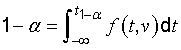defines the quantile, where f is the probability density function of t. Thus tp and t1 − α are related by p = 1 − 2α, For example, the value of the quantile t0,975, for which 1 − α = 0,975 and α = 0,025, is the same as tp(v) for p = 0,95.

# G.4   Effective degrees of freedom

G.4.1   In general, the t‑distribution will not describe the distribution of the variable (y − Y )uc(y) if u2c(y) is the sum of two or more estimated variance components u2i(y) = c2iu2(xi) (see 5.1.3), even if each xi is the estimate of a normally distributed input quantity Xi. However, the distribution of that variable may be approximated by a t‑distribution with an effective degrees of freedom veff obtained from the Welch‑Satterthwaite formula , ,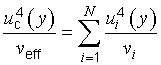(G.2a)
or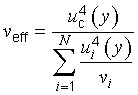(G.2b)
with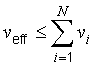(G.2c)
where u2c(y) = ∑Ni = 1u2i(y) (see 5.1.3). The expanded uncertainty Up = kpuc(y) = tp(veff)uc(y) then provides an interval Y = y ± Up having an approximate level of confidence p.

NOTE 1   If the value of veff obtained from Equation (G.2b) is not an integer, which will usually be the case in practice, the corresponding value of tp may be found from Table G.2 by interpolation or by truncating veff to the next lower integer.

NOTE 2   If an input estimate xi is itself obtained from two or more other estimates, then the value of vi to be used with u4i(y) = [c2iu2(xi)]2 in the denominator of Equation (G.2b) is the effective degrees of freedom calculated from an expression equivalent to Equation (G.2b).

NOTE 3   Depending upon the needs of the potential users of a measurement result, it may be useful, in addition to veff, to calculate and report also values for veffA and veffB, computed from Equation (G.2b) treating separately the standard uncertainties obtained from Type A and Type B evaluations. If the contributions to u2c(y) of the Type A and Type B standard uncertainties alone are denoted, respectively, by u2cA(y) and u2cB(y), the various quantities are related by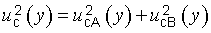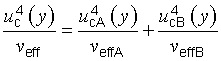EXAMPLE   Consider that Y = f(X1X2X3) = bX1X2X3 and that the estimates x1, x2, x3 of the normally distributed input quantities X1, X2, X3 are the arithmetic means of n1 = 10, n2 = 5, and n3 = 15 independent repeated observations, respectively, with relative standard uncertainties u(x1)x1 = 0,25 percent, u(x2)x2 = 0,57 percent, and u(x3)x3 = 0,82 percent. In this case, ci = ∂fXi = YXi (to be evaluated at x1, x2, x3 — see 5.1.3, Note 1), [uc(y)y]2 = ∑3i = 1[u(xi)xi]2 = (1,03 percent)2 (see Note 2 to 5.1.6), and Equation (G.2b) becomes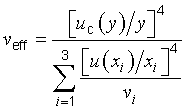Thus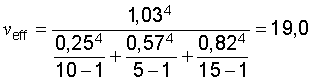The value of tp for p = 95 percent and v = 19 is, from Table G.2, t95(19) = 2,09; hence the relative expanded uncertainty for this level of confidence is U95 = 2,09 × (1,03 percent) = 2,2 percent. It may then be stated that Y = y ± U95 = y(1 ± 0,022) (y to be determined from y = bx1x2x3), or that 0,978y ≤ Y ≤ 1,022y, and that the level of confidence to be associated with the interval is approximately 95 percent.

G.4.2   In practice, uc(y) depends on standard uncertainties u(xi) of input estimates of both normally and non‑normally distributed input quantities, and the u(xi) are obtained from both frequency‑based and a priori probability distributions (that is, from both Type A and Type B evaluations). A similar statement applies to the estimate y and input estimates xi upon which y depends. Nevertheless, the probability distribution of the function t = (y − Y)uc(y) can be approximated by the t‑distribution if it is expanded in a Taylor series about its expectation. In essence, this is what is achieved, in the lowest order approximation, by the Welch‑Satterthwaite formula, Equation (G.2a) or Equation (G.2b).

The question arises as to the degrees of freedom to assign to a standard uncertainty obtained from a Type B evaluation when veff is calculated from Equation (G.2b). Since the appropriate definition of degrees of freedom recognizes that v as it appears in the t‑distribution is a measure of the uncertainty of the variance s2(z‾‾), Equation (E.7) in E.4.3 may be used to define the degrees of freedom vi,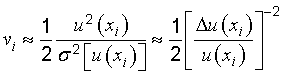(G.3)

The quantity in large brackets is the relative uncertainty of u(xi); for a Type B evaluation of standard uncertainty it is a subjective quantity whose value is obtained by scientific judgement based on the pool of available information.

EXAMPLE   Consider that one's knowledge of how input estimate xi was determined and how its standard uncertainty u(xi) was evaluated leads one to judge that the value of u(xi) is reliable to about 25 percent. This may be taken to mean that the relative uncertainty is Δu(xi)u(xi) = 0,25, and thus from Equation (G.3), vi = (0,25)−22 = 8. If instead one had judged the value of u(xi) to be reliable to only about 50 percent, then vi = 2. (See also Table E.1 in Annex E.)

G.4.3   In the discussion in 4.3 and 4.4 of Type B evaluation of standard uncertainty from an a priori probability distribution, it was implicitly assumed that the value of u(xi) resulting from such an evaluation is exactly known. For example, when u(xi) is obtained from a rectangular probability distribution of assumed half‑width a = (a+ −a)2 as in 4.3.7 and 4.4.5, u(xi) = a3‾‾ is viewed as a constant with no uncertainty because a+ and a, and thus a, are so viewed (but see 4.3.9, Note 2). This implies through Equation (G.3) that vi → ∞ or 1vi → 0, but it causes no difficulty in evaluating Equation (G.2b). Further, assuming that vi → ∞ is not necessarily unrealistic; it is common practice to choose a and a+ in such a way that the probability of the quantity in question lying outside the interval a to a+ is extremely small.

# G.5   Other considerations

G.5.1   An expression found in the literature on measurement uncertainty and often used to obtain an uncertainty that is intended to provide an interval with a 95 percent level of confidence may be written as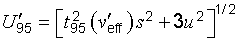(G.4)

Here t95(veff) is taken from the t‑distribution for veff degrees of freedom and p = 95 percent; veff is the effective degrees of freedom calculated from the Welch‑Satterthwaite formula [Equation (G.2b)] taking into account only those standard uncertainty components si that have been evaluated statistically from repeated observations in the current measurement; s2 = ∑c2is2i; ci ≡ ∂fxi; and u2 = ∑u2j(y) = ∑c2j(a2j3) accounts for all other components of uncertainty, where +aj and aj are the assumed exactly known upper and lower bounds of Xj relative to its best estimate xj (that is, xj − aj ≤ Xj ≤ xj + aj).

NOTE   A component based on repeated observations made outside the current measurement is treated in the same way as any other component included in u2. Hence, in order to make a meaningful comparison between Equation (G.4) and Equation (G.5) of the following subclause, it is assumed that such components, if present, are negligible.

G.5.2   If an expanded uncertainty that provides an interval with a 95 percent level of confidence is evaluated according to the methods recommended in G.3 and G.4, the resulting expression in place of Equation (G.4) is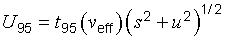(G.5)
where veff is calculated from Equation (G.2b) and the calculation includes all uncertainty components.

In most cases, the value of U95 from Equation (G.5) will be larger than the value of U95 from Equation (G.4), if it is assumed that in evaluating Equation (G.5), all Type B variances are obtained from a priori rectangular distributions with half‑widths that are the same as the bounds aj used to compute u2 of Equation (G.4). This may be understood by recognizing that, although t95(veff) will in most cases be somewhat larger than t95(veff), both factors are close to 2; and in Equation (G.5) u2 is multiplied by t2p(veff) ≈ 4 while in Equation (G.4) it is multiplied by 3. Although the two expressions yield equal values of U95 and U95 for u2 ≪ s2, U95 will be as much as 13 percent smaller than U95 if u2 ≫ s2. Thus in general, Equation (G.4) yields an uncertainty that provides an interval having a smaller level of confidence than the interval provided by the expanded uncertainty calculated from Equation (G.5).

NOTE 1   In the limits u2s2 → ∞ and veff → ∞, U95 → 1,732u while U95 → 1,960u. In this case, U95 provides an interval having only a 91,7 percent level of confidence, while U95 provides a 95 percent interval. This case is approximated in practice when the components obtained from estimates of upper and lower bounds are dominant, large in number, and have values of u2j(y) = c2ja2j3 that are of comparable size.

NOTE 2   For a normal distribution, the coverage factor k = 3‾‾ ≈ 1,732 provides an interval with a level of confidence p = 91,673... percent. This value of p is robust in the sense that it is, in comparison with that of any other value, optimally independent of small deviations of the input quantities from normality.

G.5.3   Occasionally an input quantity Xi is distributed asymmetrically — deviations about its expected value of one sign are more probable than deviations of the opposite sign (see 4.3.8). Although this makes no difference in the evaluation of the standard uncertainty u(xi) of the estimate xi of Xi, and thus in the evaluation of uc(y), it may affect the calculation of U.

It is usually convenient to give a symmetric interval, Y = y ± U, unless the interval is such that there is a cost differential between deviations of one sign over the other. If the asymmetry of Xi causes only a small asymmetry in the probability distribution characterized by the measurement result y and its combined standard uncertainty uc(y), the probability lost on one side by quoting a symmetric interval is compensated by the probability gained on the other side. The alternative is to give an interval that is symmetric in probability (and thus asymmetric in U): the probability that Y lies below the lower limit y − U is equal to the probability that Y lies above the upper limit y + U+. But in order to quote such limits, more information than simply the estimates y and uc(y) [and hence more information than simply the estimates xi and u(xi) of each input quantity Xi] is needed.

G.5.4   The evaluation of the expanded uncertainty Up given here in terms of uc(y), veff, and the factor tp(veff) from the t‑distribution is only an approximation, and it has its limitations. The distribution of (y − Y)uc(y) is given by the t‑distribution only if the distribution of Y is normal, the estimate y and its combined standard uncertainty uc(y) are independent, and if the distribution of u2c(y) is a χ2 distribution. The introduction of veff, Equation (G.2b), deals only with the latter problem, and provides an approximately χ2 distribution for u2c(y); the other part of the problem, arising from the non‑normality of the distribution of Y, requires the consideration of higher moments in addition to the variance.

# G.6   Summary and conclusions

G.6.1   The coverage factor kp that provides an interval having a level of confidence p close to a specified level can only be found if there is extensive knowledge of the probability distribution of each input quantity and if these distributions are combined to obtain the distribution of the output quantity. The input estimates xi and their standard uncertainties u(xi) by themselves are inadequate for this purpose.

G.6.2   Because the extensive computations required to combine probability distributions are seldom justified by the extent and reliability of the available information, an approximation to the distribution of the output quantity is acceptable. Because of the Central Limit Theorem, it is usually sufficient to assume that the probability distribution of (y − Y )uc(y) is the t‑distribution and take kp = tp(veff), with the t‑factor based on an effective degrees of freedom veff of uc(y) obtained from the Welch‑Satterthwaite formula, Equation (G.2b).

G.6.3   To obtain veff from Equation (G.2b) requires the degrees of freedom vi for each standard uncertainty component. For a component obtained from a Type A evaluation, vi is obtained from the number of independent repeated observations upon which the corresponding input estimate is based and the number of independent quantities determined from those observations (see G.3.3). For a component obtained from a Type B evaluation, vi is obtained from the judged reliability of the value of that component [see G.4.2 and Equation (G.3)].

G.6.4   Thus the following is a summary of the preferred method of calculating an expanded uncertainty Up = kpuc(y) intended to provide an interval Y = y ± Up that has an approximate level of confidence p:

1. Obtain y and uc(y) as described in Clauses 4 and 5.
2. Compute veff from the Welch‑Satterthwaite formula, Equation (G.2b) (repeated here for easy reference)(G.2b)

If u(xi) is obtained from a Type A evaluation, determine vi as outlined in G.3.3. If u(xi) is obtained from a Type B evaluation and it can be treated as exactly known, which is often the case in practice, vi → ∞; otherwise, estimate vi from Equation (G.3).

3. Obtain the t‑factor tp(veff) for the desired level of confidence p from Table G.2. If veff is not an integer, either interpolate or truncate veff to the next lower integer.
4. Take kp = tp(veff) and calculate Up = kpuc(y).

G.6.5   In certain situations, which should not occur too frequently in practice, the conditions required by the Central Limit Theorem may not be well met and the approach of G.6.4 may lead to an unacceptable result. For example, if uc(y) is dominated by a component of uncertainty evaluated from a rectangular distribution whose bounds are assumed to be exactly known, it is possible [if tp(veff) > 3‾‾] that y + Up and y − Up, the upper and lower limits of the interval defined by Up, could lie outside the bounds of the probability distribution of the output quantity Y. Such cases must be dealt with on an individual basis but are often amenable to an approximate analytic treatment (involving, for example, the convolution of a normal distribution with a rectangular distribution ).

G.6.6   For many practical measurements in a broad range of fields, the following conditions prevail:

• the estimate y of the measurand Y is obtained from estimates xi of a significant number of input quantities Xi that are describable by well‑behaved probability distributions, such as the normal and rectangular distributions;
• the standard uncertainties u(xi) of these estimates, which may be obtained from either Type A or Type B evaluations, contribute comparable amounts to the combined standard uncertainty uc(y) of the measurement result y;
• the linear approximation implied by the law of propagation of uncertainty is adequate (see 5.1.2 and E.3.1);
• the uncertainty of uc(y) is reasonably small because its effective degrees of freedom veff has a significant magnitude, say greater than 10.

Under these circumstances, the probability distribution characterized by the measurement result and its combined standard uncertainty can be assumed to be normal because of the Central Limit Theorem; and uc(y) can be taken as a reasonably reliable estimate of the standard deviation of that normal distribution because of the significant size of veff. Then, based on the discussion given in this annex, including that emphasizing the approximate nature of the uncertainty evaluation process and the impracticality of trying to distinguish between intervals having levels of confidence that differ by one or two percent, one may do the following:

• adopt k = 2 and assume that U = 2uc(y) defines an interval having a level of confidence of approximately 95 percent;
or, for more critical applications,
• adopt k = 3 and assume that U = 3uc(y) defines an interval having a level of confidence of approximately 99 percent.

Although this approach should be suitable for many practical measurements, its applicability to any particular measurement will depend on how close k = 2 must be to t95(veff) or k = 3 must be to t99(veff); that is, on how close the level of confidence of the interval defined by U = 2uc(y) or U = 3uc(y) must be to 95 percent or 99 percent, respectively. Although for veff = 11, k = 2 and k = 3 underestimate t95(11) and t99(11) by only about 10 percent and 4 percent, respectively (see Table G.2), this may not be acceptable in some cases. Further, for all values of veff somewhat larger than 13, k = 3 produces an interval having a level of confidence larger than 99 percent. (See Table G.2, which also shows that for veff → ∞ the levels of confidence of the intervals produced by k = 2 and k = 3 are 95,45 percent and 99,73 percent, respectively). Thus, in practice, the size of veff and what is required of the expanded uncertainty will determine whether this approach can be used.

Table G.2 — Value of tp(v) from the t‑distribution for degrees of freedom v that defines an interval tp(v) to +tp(v) that encompasses the fraction p of the distribution
Degrees of freedom Fraction p in percent
v 68,27a) 90 95 95,45a) 99 99,73a)

a)   For a quantity z described by a normal distribution with expectation μz and standard deviation σ, the interval μz ±  encompasses p = 68,27 percent, 95,45 percent and 99,73 percent of the distribution for k = 1, 2 and 3, respectively.

1 1,84 6,31 12,71 13,97 63,66 235,80
2 1,32 2,92 4,30 4,53 9,92 19,21
3 1,20 2,35 3,18 3,31 5,84 9,22
4 1,14 2,13 2,78 2,87 4,60 6,62
5 1,11 2,02 2,57 2,65 4,03 5,51

6 1,09 1,94 2,45 2,52 3,71 4,90
7 1,08 1,89 2,36 2,43 3,50 4,53
8 1,07 1,86 2,31 2,37 3,36 4,28
9 1,06 1,83 2,26 2,32 3,25 4,09
10 1,05 1,81 2,23 2,28 3,17 3,96

11 1,05 1,80 2,20 2,25 3,11 3,85
12 1,04 1,78 2,18 2,23 3,05 3,76
13 1,04 1,77 2,16 2,21 3,01 3,69
14 1,04 1,76 2,14 2,20 2,98 3,64
15 1,03 1,75 2,13 2,18 2,95 3,59

16 1,03 1,75 2,12 2,17 2,92 3,54
17 1,03 1,74 2,11 2,16 2,90 3,51
18 1,03 1,73 2,10 2,15 2,88 3,48
19 1,03 1,73 2,09 2,14 2,86 3,45
20 1,03 1,72 2,09 2,13 2,85 3,42

25 1,02 1,71 2,06 2,11 2,79 3,33
30 1,02 1,70 2,04 2,09 2,75 3,27
35 1,01 1,70 2,03 2,07 2,72 3,23
40 1,01 1,68 2,02 2,06 2,70 3,20
45 1,01 1,68 2,01 2,06 2,69 3,18

50 1,01 1,68 2,01 2,05 2,68 3,16
100 1,005 1,660 1,984 2,025 2,626 3,077
1,000 1,645 1,960 2,000 2,576 3,000